International
Tables for
Crystallography
Volume F
Crystallography of biological macromolecules
Edited by M. G. Rossmann and E. Arnold

International Tables for Crystallography (2006). Vol. F, ch. 18.5, p. 409   | 1 | 2 |

## Section 18.5.5. Approximate methods

D. W. J. Cruickshanka*

aChemistry Department, UMIST, Manchester M60 1QD, England
Correspondence e-mail: dwj_cruickshank@email.msn.com

### 18.5.5. Approximate methods

| top | pdf |

#### 18.5.5.1. Block calculations

| top | pdf |

The full-matrix inversions described in the previous section require massive calculations. The length of the calculations is more a matter of the order of the matrix, i.e., the number of parameters, than of the number of observations. When restraints are applied, it is the diffraction-cum-restraints full matrix which should be inverted.

With the increasing power of computers and more efficient algorithms (e.g. Tronrud, 1999; Murshudov et al., 1999), a final full matrix should be computed and inverted much more regularly – and not just for high-resolution analyses. Low-resolution analyses have a need, beyond the indications given by B values, to identify through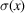estimates their regions of tolerable and less tolerable precision.

If full-matrix calculations are impractical, partial schemes can be suggested. As far back as 1973, Watenpaugh et al. (1973), in a study of rubredoxin at 1.5 Å resolution, effectively inverted the diffraction full matrix in 200 parameter blocks to obtain individual s.u.'s. A similar scheme for restrained refinements could also use overlapping large blocks. A minimal block scheme in refinements of any resolution is to calculate blocks for each residue and for the block interactions between successive residues. The inversion process could then use the matrices in running groups of three successive residues, taking only the inverted elements for the central residue as the estimates of its variances and covariances.

For low-resolution analyses with very large numbers of atoms, it might be sufficient to gain a general idea of the behaviour ofas a function of B by computing a limited number of blocks for representative or critical groups of residues. The parameters used in the blocks should include the B's, since atomic images overlap at low resolution, thus correlating the position of one atom with the displacement parameters of its neighbours.

#### 18.5.5.2. The modified Fourier method

| top | pdf |

In the simplest form of the Fourier-map approach to centrosymmetric high-resolution structures, atomic positions are given by the maxima of the observed electron density. The uncertainty of such a position may be estimated as the uncertainty in the slope function (first derivative) divided by the curvature (second derivative) at the peak (Cruickshank, 1949a), i.e.,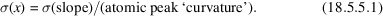However, atomic positions are affected by finite-series and peak-overlapping effects.

Hence, more generally, atomic positions may be determined by the requirement that the slope of the difference map at the position of atom r should be zero, or equivalently that the slopes at atom r of the observed and calculated electron densities should be equal. As a criterion this becomes the basis of the modified Fourier method (Cruickshank, 1952, 1959, 1999; Bricogne, 2001, Section 1.3.4.4.7.5), which, like the least-squares method, is applicable whether or not the atomic peaks are resolved and is applicable to noncentrosymmetric structures. For refinement, a set of n simultaneous linear equations are involved, analogous to the normal equations of least squares. Their right-hand sides are the slopes of the difference map at the trial atomic positions.

The diagonal elements of the matrix, for coordinateof an atom with Debye B value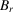, are approximately equal to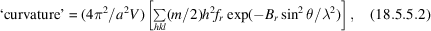whereor 2 for acentric or centric reflections. The summation is over all independent planes and their symmetry equivalents. Strictly speaking, (18.5.5.2)is a curvature only for centrosymmetric structures.

In the modified Fourier method,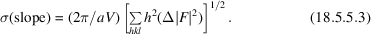This is simply an estimate of the r.m.s. uncertainty at a general position (Cruickshank & Rollett, 1953) in the slope of the difference map, i.e., the r.m.s. uncertainty on the right-hand side of the modified Fourier method.is then given by (18.5.5.1), using (18.5.5.3)and (18.5.5.2).

#### 18.5.5.3. Application of the modified Fourier method

| top | pdf |

An extreme example of an apparently successful gross approximation to protein precision is represented by Daopin et al.'s (1994)treatment of two independent determinations (at 1.8 and 1.95 Å) of the structure of TGF-β2. They reported that the modified Fourier-map formulae given in Section 18.5.5.2yielded a quite good description of the B dependence of the positional differences between the two independent determinations. However, there is a formal difficulty about this application. Equation (18.5.5.1)derives from a diffraction-data-only approach, whereas the two structures were determined from restrained refinements. Even though the TNT restraint parameters and weights may have been the same in both refinements, it is slightly surprising that (18.5.5.1)should have worked well.

Equation (18.5.2.1)requires the summation of various series over all (hkl) observations; such calculations are not customarily provided in protein programs. However, due to the fundamental similarities between Fourier and least-squares methods demonstrated by Cochran (1948), Cruickshank (1949b, 1952, 1959), and Cruickshank & Robertson (1953), closely similar estimates of the precision of individual atoms can be obtained from the reciprocal of the diagonal elements of the diffraction-data-only least-squares matrix. These elements will often have been calculated already within the protein refinement programs, but possibly never output. Such estimates could be routinely available.

Between approximations using largish blocks and those using only the reciprocals of diagonal terms, a whole variety of intermediate approximations involving some off-diagonal terms could be envisaged.

Whatever method is used to estimate uncertainties, it is essential to distinguish between coordinate uncertainty, e.g.,, and position uncertainty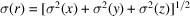.

The remainder of this chapter discusses two rough-and-ready indicators of structure precision: the diffraction-component precision index (DPI) and Luzzati plots.

### References

Bricogne, G. (2001). Fourier transforms in crystallography: theory, algorithms, and applications. In International tables for crystallography, Vol. B, edited by U. Shmueli, ch. 1.3. Dordrecht: Kluwer Academic Publishers.Google Scholar
Cochran, W. (1948). The Fourier method of crystal-structure analysis. Acta Cryst. 1, 138–142.Google Scholar
Cruickshank, D. W. J. (1949a). The accuracy of electron-density maps in X-ray analysis with special reference to dibenzyl. Acta Cryst. 2, 65–82.Google Scholar
Cruickshank, D. W. J. (1949b). The accuracy of atomic coordinates derived by least-squares or Fourier methods. Acta Cryst. 2, 154–157.Google Scholar
Cruickshank, D. W. J. (1952). On the relations between Fourier and least-squares methods of structure determination. Acta Cryst. 5, 511–518.Google Scholar
Cruickshank, D. W. J. (1959). Statistics. In International tables for X-ray crystallography, Vol. 2, edited by J. S. Kasper & K. Lonsdale, pp. 84–98. Birmingham: Kynoch Press.Google Scholar
Cruickshank, D. W. J. (1999). Remarks about protein structure precision. Acta Cryst. D55, 583–601.Google Scholar
Cruickshank, D. W. J. & Robertson, A. P. (1953). The comparison of theoretical and experimental determinations of molecular structures, with applications to naphthalene and anthracene. Acta Cryst. 6, 698–705.Google Scholar
Cruickshank, D. W. J. & Rollett, J. S. (1953). Electron-density errors at special positions. Acta Cryst. 6, 705–707.Google Scholar
Daopin, S., Davies, D. R., Schlunegger, M. P. & Grütter, M. G. (1994). Comparison of two crystal structures of TGF-β2: the accuracy of refined protein structures. Acta Cryst. D50, 85–92.Google Scholar
Murshudov, G. N., Vagin, A. A., Lebedev, A., Wilson, K. S. & Dodson, E. J. (1999). Efficient anisotropic refinement of macromolecular structures using FFT. Acta Cryst. D55, 247–255.Google Scholar
Tronrud, D. E. (1999). The efficient calculation of the normal matrix in least-squares refinement of macromolecular structures. Acta Cryst. A55, 700–703.Google Scholar
Watenpaugh, K. D., Sieker, L. C., Herriott, J. R. & Jensen, L. H. (1973). Refinement of the model of a protein: rubredoxin at 1.5 Å resolution. Acta Cryst. B29, 943–956.Google Scholar